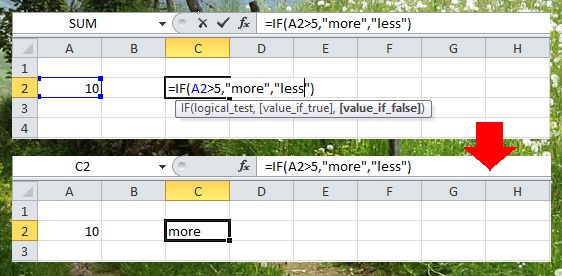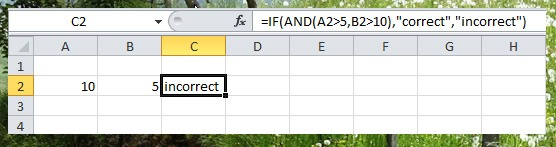# Logical Functions

One of the best thing in Excel is to compare data in different cells, using logical functions you can easily compare each cell that you want to compare and get the output that you need.

In this lesson you will learn the basic Excel logical functions IF, AND and OR.

IF Function

IF Function is used to check if the condition is met and return the first value if TRUE and another value if FALSEThe example above return “more” because the logical test is true which is 10>5.

There are three arguments in IF Function

• logical_test – The condition to test
• value_if_true – Value to return if the logical_test is true
• value_if_true – Value to return if the logical_test is false

AND Function

AND Functions use to test conditions and return TRUE if all the conditions are true and FALSE if any of the conditions are false.In the example above, the result is “Incorrect” because the value in B2 which is 5 is not greater than 10. Since in AND functions the condition must be all true, the IF function returns Incorrect.

OR Function

The OR Function returns TRUE if the any of the conditions is true and return FALSE if all conditions are false.Since we use OR function in the example above which the same conditions when we use AND functions, the return value is “Correct”. Any of the conditions is true the If functions return true.

#### One Response

1.Mhelvin